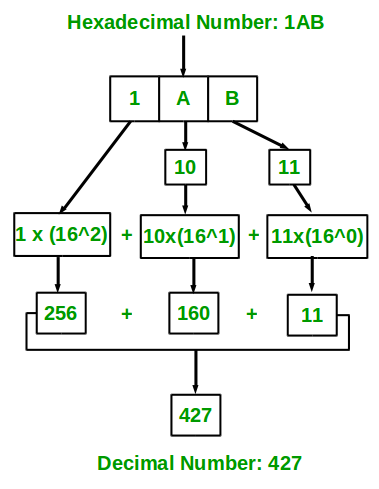Related Articles
Java Program For Hexadecimal to Decimal Conversion
• Last Updated : 09 Mar, 2021

Given a Hexadecimal number N, convert N into an equivalent decimal number i.e convert the number with base value 16 to base value 10. The decimal number system uses 10 digits 0-9 and the Hexadecimal number system uses 0-9, A-F to represent any numeric value.

Illustration:

```Input : 1AB
Output: 427

Input : 1A
Output: 26```

Approach:

1. The idea is to extract the digits of a given hexadecimal number starting from the rightmost digit.
2. Keep a variable ‘dec_value’.
3. At the time of extracting digits from the hexadecimal number, multiply the digit with the proper base (Power of 16) and add it to the above variable taken that is ‘dec_value’.
4. In the end, the variable ‘dec_value’ will store the required decimal number.convert hexadecimal number ( 1AB ) to equivalent decimal value

Implementation:

Example

## Java

 `// Java program to convert Hexadecimal to Decimal Number`` ` `// Importing input output classes``import` `java.io.*;`` ` `// Main class``class` `GFG {`` ` `    ``// Method``    ``// To convert hexadecimal to decimal``    ``static` `int` `hexadecimalToDecimal(String hexVal)``    ``{``        ``// Storing the length of the``        ``int` `len = hexVal.length();`` ` `        ``// Initializing base value to 1, i.e 16^0``        ``int` `base = ``1``;`` ` `        ``// Initially declaring and initializing``        ``// decimal value to zero``        ``int` `dec_val = ``0``;`` ` `        ``// Extracting characters as``        ``// digits from last character`` ` `        ``for` `(``int` `i = len - ``1``; i >= ``0``; i--) {`` ` `            ``// Condition check``            ``// Case 1``            ``// If character lies in '0'-'9', converting``            ``// it to integral 0-9 by subtracting 48 from``            ``// ASCII value``            ``if` `(hexVal.charAt(i) >= ``'0'``                ``&& hexVal.charAt(i) <= ``'9'``) {``                ``dec_val += (hexVal.charAt(i) - ``48``) * base;`` ` `                ``// Incrementing base by power``                ``base = base * ``16``;``            ``}`` ` `            ``// Case 2``            ``// if case 1 is bypassed`` ` `            ``// Now, if character lies in 'A'-'F' ,``            ``// converting it to integral 10 - 15 by``            ``// subtracting 55 from ASCII value``            ``else` `if` `(hexVal.charAt(i) >= ``'A'``                     ``&& hexVal.charAt(i) <= ``'F'``) {``                ``dec_val += (hexVal.charAt(i) - ``55``) * base;`` ` `                ``// Incrementing base by power``                ``base = base * ``16``;``            ``}``        ``}`` ` `        ``// Returning the decimal value``        ``return` `dec_val;``    ``}`` ` `    ``// Method 2``    ``// Main driver method``    ``public` `static` `void` `main(String[] args)``    ``{``        ``// Custom input hexadecimal number to be``        ``// converted into decimal number``        ``String hexNum = ``"1A"``;`` ` `        ``// Calling the above method to convert and``        ``// alongside printing the hexadecimal number``        ``System.out.println(hexadecimalToDecimal(hexNum));``    ``}``}`
Output
`26`

Attention reader! Don’t stop learning now. Get hold of all the important Java Foundation and Collections concepts with the Fundamentals of Java and Java Collections Course at a student-friendly price and become industry ready. To complete your preparation from learning a language to DS Algo and many more,  please refer Complete Interview Preparation Course.

My Personal Notes arrow_drop_up